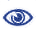# The Prague Stringology Conference 2006

Efficient Algorithms for (δ, γ, α)-Matching

 Abstract: We propose new algorithms for (δ, γ, α)-matching. In this string matching problem we are given a pattern P=p0p1...pm-1 and a text T=t0t1...tn-1 over some integer alphabet Σ = {0...σ-1}. The pattern symbol pi matches the text symbol tj  iff  |pi-tj| ≤ δ. The pattern P (δ,γ)-matches some text substring tj...tj+m-1  iff  for all i it holds that |pi-tj+i| ≤ δ and ∑|pi-tj+i| ≤ γ. Finally, in (δ, γ, α)-matching we also permit at most α length gaps (text substrings) between each matching text symbol. The only known previous algorithm runs in O(mn) time. We give several algorithms that improve the average case up to O(n) for small α, and the worst case to O(min{mn,|M|α}) or O(mn log (γ/w)), where M = {(i,j);  |pi-tj| ≤ δ} and w is the number of bits in a machine word. We conclude with experimental results showing that the algorithms are very efficient in practice.

 Download paper:PostScript PDF BibTeX reference
 Download presentation: